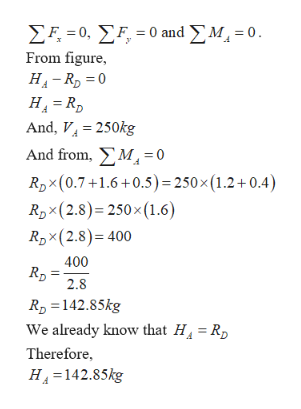Question
18 views

The frame shown supports a mass of m=250kg mass suspended from point F. The frame is supported by a pin connection at Point A and a roller at Point D. Internal pin connections are located at Points B, C, and E. The dimensions of the frame are h1=0.7m, h2=1.6m, h3=0.5m, L1=1.2m, L2=0.4m. Determine:

• The vertical and horizontal components of the reaction force at Point A.
• The vertical and horizontal components of the force at Pin C.
• The reaction force at Point D.
check_circle

Step 1

Given:

The frame having following values.

Step 2

Calculation:

Now, draw the free body diagram for the given frame is

Step 3
1. Calculating vertical and horizontal components o...help_outlineImage TranscriptioncloseΣΗ-0, ΣF -0 md ΣΜ,-0. From figure HA-RD 0 H RD And, V250kg And from,M= 0 RDX(0.7+1.6+0.5)= 250 x (1.2+0.4) RDx (2.8) 250x (1.6 RDx(2.8) 400 400 RD 2.8 RD 142.85kg We already know that H4 R Therefore H4 142.85kg fullscreen

### Want to see the full answer?

See Solution

#### Want to see this answer and more?

Solutions are written by subject experts who are available 24/7. Questions are typically answered within 1 hour.*

See Solution
*Response times may vary by subject and question.
Tagged in

### Mechanics